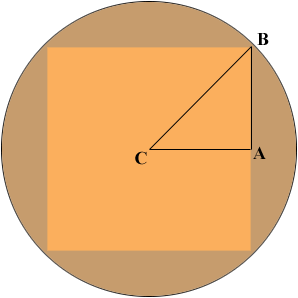SEARCH HOMEMath Central Quandaries & QueriesQuestion from Middle, a student: what is the perimeter of a square inscribed in a circle of radius 5.0 inches?Draw a diagram!C is the centre of the circle so the length of CB is 5.0 inches. Suppose the length of a side of the square is s inches. What i the length of CA? What is the length of AB? What does Pythagoras theorem tell you?

PennyMath Central is supported by the University of Regina and The Pacific Institute for the Mathematical Sciences.